## 39:06 The magnetic field 0.02 m from a wire is 0.1 T. What is the magnitude of the magnetic field 0.01 m from the same wire?

Question

39:06
The magnetic field 0.02 m from a wire is 0.1 T. What is the magnitude of the magnetic field 0.01 m from the same wire?

in progress 0
5 months 2021-08-13T01:33:34+00:00 1 Answers 0 views 0

The magnetic field 0.01m from the wire is 0.2T.

Explanation:

The magnetic field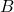at a distance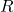due to a wire carrying current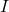is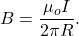Now, let us call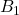the magnetic field at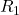and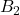the magnetic field at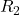: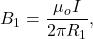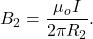Dividingbywe get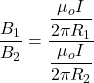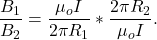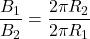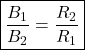Now we put in the numbers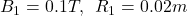and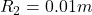to get: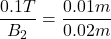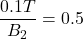solving forwe get: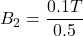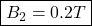which is the magnetic field at 0.01 meters from the wire.